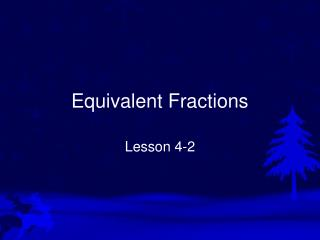DownloadDownload PresentationEquivalent Fractions

# Equivalent Fractions

Download Presentation## Equivalent Fractions

- - - - - - - - - - - - - - - - - - - - - - - - - - - E N D - - - - - - - - - - - - - - - - - - - - - - - - - - -
##### Presentation Transcript

1. Equivalent Fractions Lesson 4-2

2. Vocabulary Equivalent fractions are fractions that name the same amount. 2 4 = 8 4

3. Creating Equivalent Fractions • Multiply the numerator and denominator by the same number. • Divide the numerator and denominator by the same number (it has to be a common factor to work with division) We can choose any number to multiply by. Let’s multiply by 2. 3 x 2 6 So, 3/5 is equivalent to 6/10. = x 2 10 5

4. If you have larger numbers, you can make equivalent fractions using division. Divide by a common factor. 4 28 ÷7 In this example, we can divide both numbers by 7. = 5 ÷7 35 28/35 is equivalent to 4/5.

5. Fractions in Simplest Form Fractions are in simplest form when the numerator and denominator do not have any common factors besides 1. Examples of fractions that are in simplest form: 4 2 3 8 5 11

6. Writing Fractions in Simplest Form. • Find the greatest common factor (GCF) of the numerator and denominator. • Divide both numbers by the GCF.

7. Example: 5 20 ÷ 4 = Simplest Form 7 ÷ 4 28 20: 1, 2, 4, 5, 10, 20 20 28 28: 1, 2, 4, 7, 14, 28 1 x 20 2 x 10 4 x 5 1 x 28 2 x 14 4 x 7 Common Factors: 1, 2, 4 GCF: 4 We will divide by 4.

8. Practice: Put each fraction in its simplest form • 9) 7/28 • GCF: 7  divide both by 7 1/4 • 10) 9/15 • GCF: 3  divide both by 3 3/5 • 11) 10/42 • GCF: 2  divide both by 2 5/21 • 12) 12/42 • GCF: 6  divide both by 6 2/7

9. Homework Time Booklet Page 59 9 18